# Phys 2120 Spring 2017 A Dzyubenko Chapter 17

• Slides: 51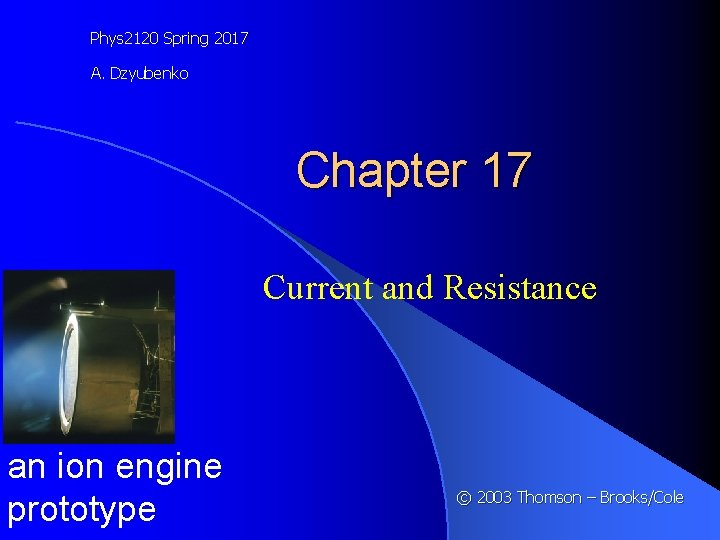Phys 2120 Spring 2017 A. Dzyubenko Chapter 17 Current and Resistance an ion engine prototype © 2003 Thomson – Brooks/ColeElectric Current Positive charges as current carriers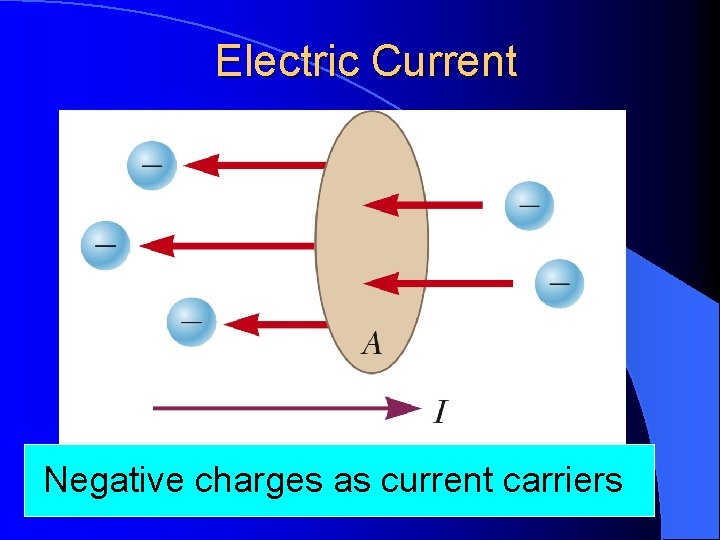Electric Current Negative charges as current carriersElectric Current l Whenever electric charges of like signs move, an electric current is said to exist l The current is the rate at which the charge flows through this surface l l The Look at the charges flowing perpendicularly to a surface of area A SI unit of current is Ampere (A) l 1 A = 1 C/sElectric Current l The direction of current flow is the direction positive charge would flow – This is known as conventional current flow l In a common conductor, such as copper, the current is due to the motion of the negatively charged electrons l It is common to refer to a moving charge as a mobile charge carrier l A charge carriers can be positive or negative or bothQUICK QUIZ 17. 1 Consider positive and negative charges moving horizontally through the four regions in Figure 17. 2. Rank the currents in these four regions, from lowest to highest.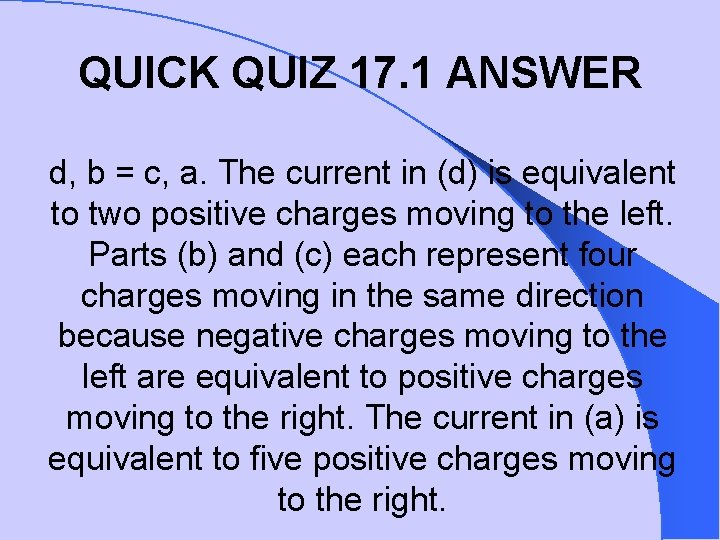QUICK QUIZ 17. 1 ANSWER d, b = c, a. The current in (d) is equivalent to two positive charges moving to the left. Parts (b) and (c) each represent four charges moving in the same direction because negative charges moving to the left are equivalent to positive charges moving to the right. The current in (a) is equivalent to five positive charges moving to the right.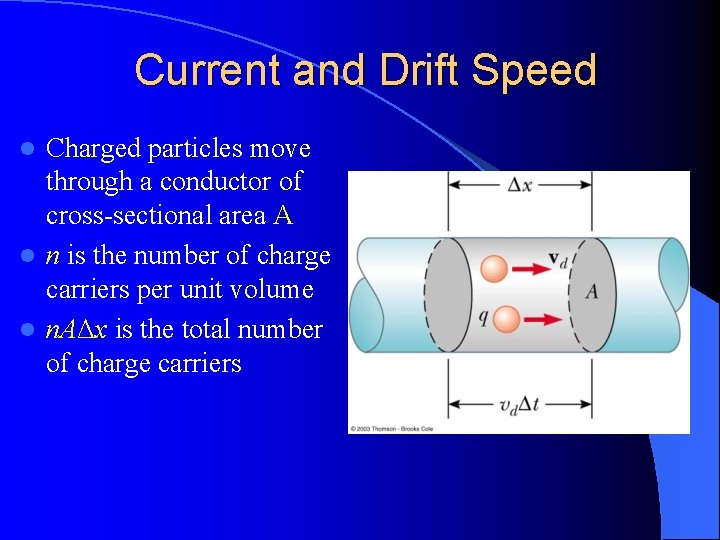Current and Drift Speed Charged particles move through a conductor of cross-sectional area A l n is the number of charge carriers per unit volume l n. AΔx is the total number of charge carriers l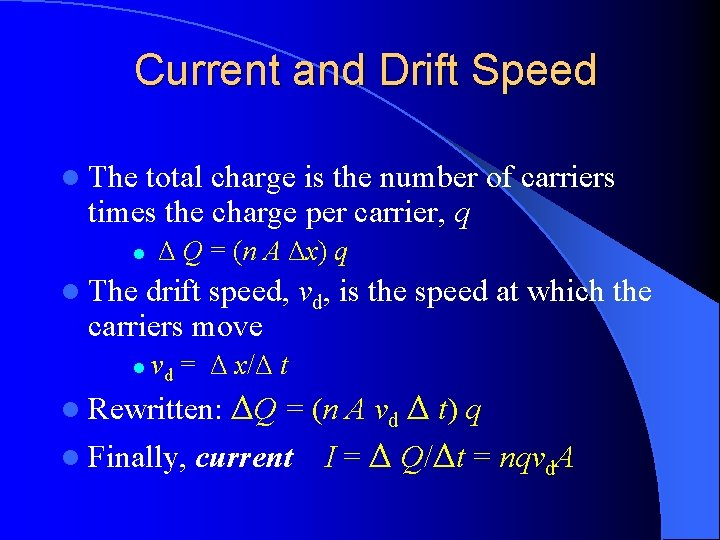Current and Drift Speed l The total charge is the number of carriers times the charge per carrier, q l Δ Q = (n A Δx) q l The drift speed, vd, is the speed at which the carriers move l vd = Δ x/Δ t ΔQ = (n A vd Δ t) q l Finally, current I = Δ Q/Δt = nqvd. A l Rewritten: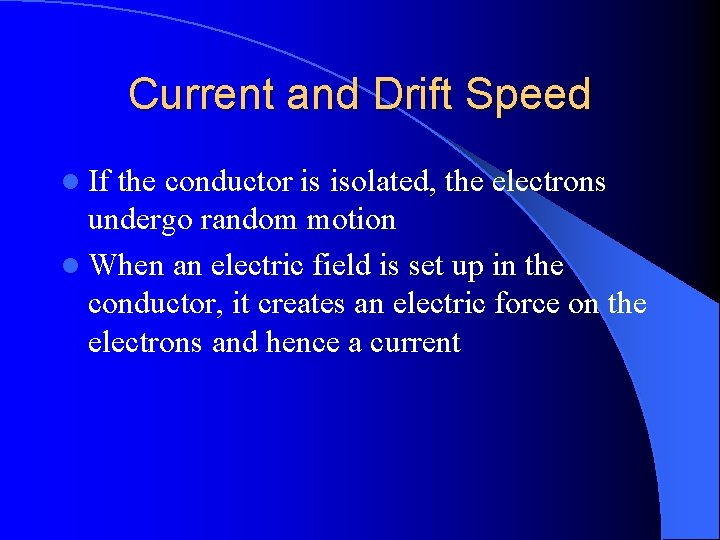Current and Drift Speed l If the conductor is isolated, the electrons undergo random motion l When an electric field is set up in the conductor, it creates an electric force on the electrons and hence a current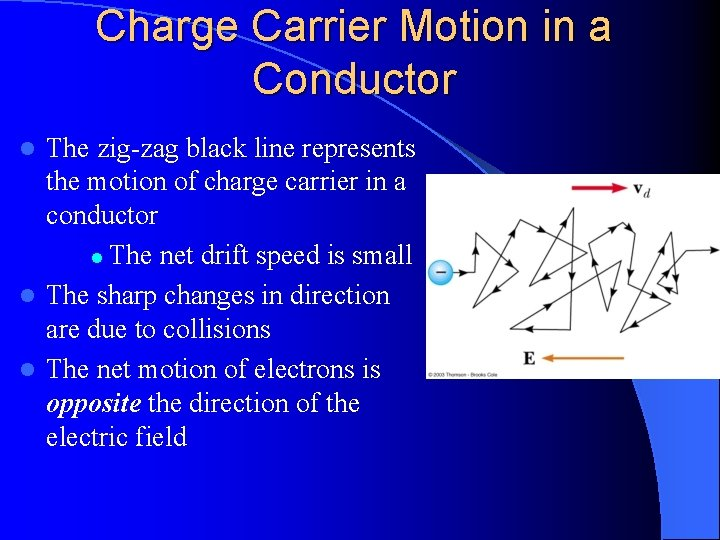Charge Carrier Motion in a Conductor The zig-zag black line represents the motion of charge carrier in a conductor l The net drift speed is small l The sharp changes in direction are due to collisions l The net motion of electrons is opposite the direction of the electric field lElectrons in a Circuit l The drift speed is much smaller than the average speed between collisions l When a circuit is completed, the electric field travels with a speed close to the speed of light l Although the drift speed is on the order of 10 -4 m/s the effect of the electric field is felt on the order of 108 m/s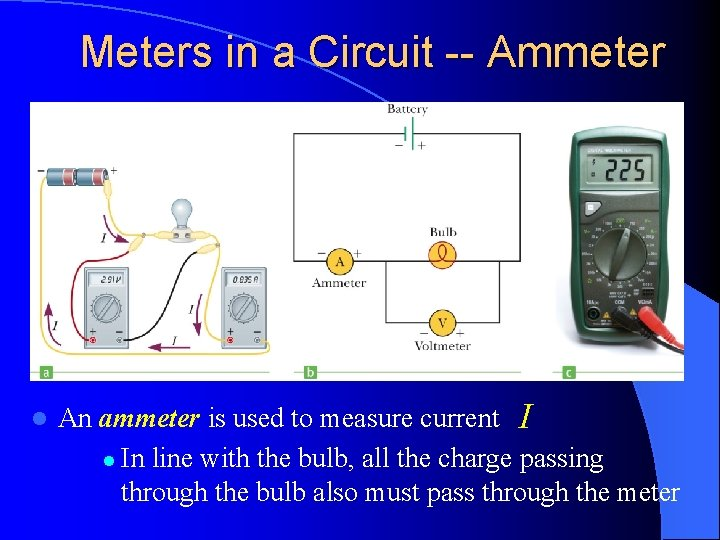Meters in a Circuit -- Ammeter l An ammeter is used to measure current I l In line with the bulb, all the charge passing through the bulb also must pass through the meter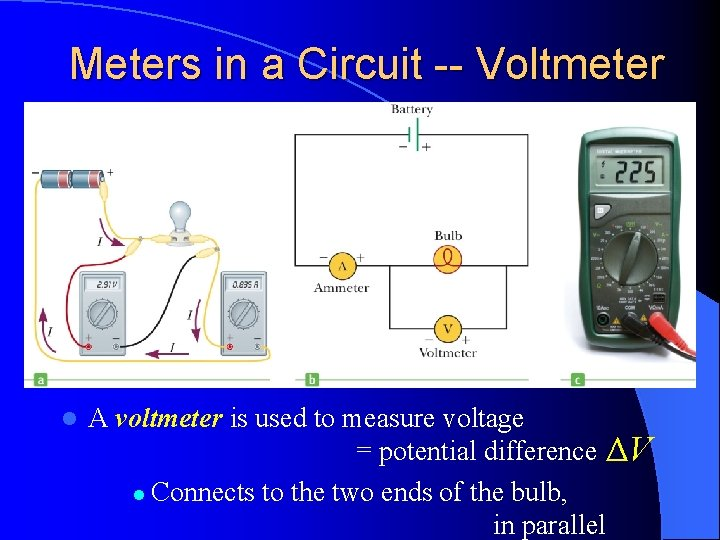Meters in a Circuit -- Voltmeter l A voltmeter is used to measure voltage = potential difference ΔV l Connects to the two ends of the bulb, in parallel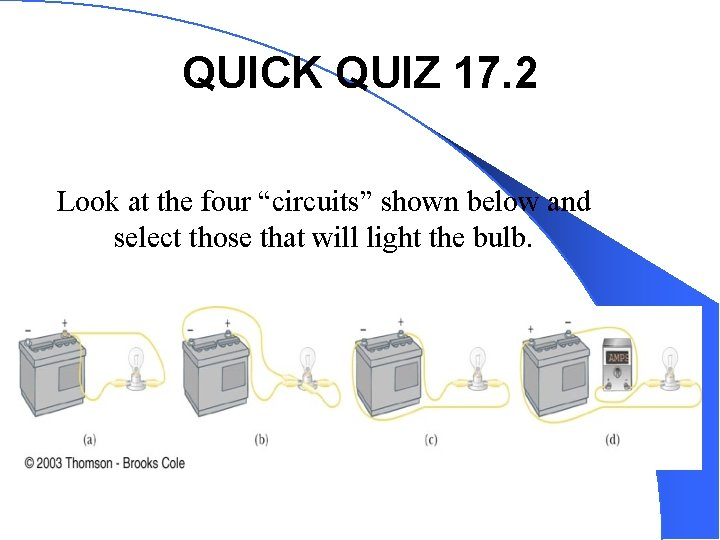QUICK QUIZ 17. 2 Look at the four “circuits” shown below and select those that will light the bulb.QUICK QUIZ 17. 2 ANSWER (c) and (d) Neither circuit (a) nor circuit (b) applies a difference in potential across the bulb. Circuit (a) has both lead wires connected to the same battery terminal. Circuit (b) has a low resistance path (a “short”) between the two battery terminals as well as between the bulb terminals.Resistance l In a conductor, the voltage applied across the ends of the conductor is proportional to the current through the conductor l The constant of proportionality is the resistance of the conductorResistance l Units l of resistance are Ohms (Ω) 1Ω=1 V/A l Resistance in a circuit arises due to collisions between the electrons carrying the current with the fixed atoms inside the conductor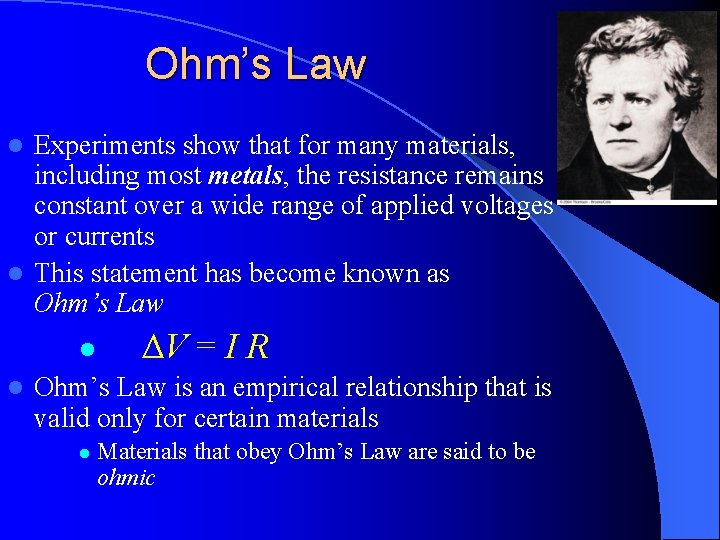Ohm’s Law Experiments show that for many materials, including most metals, the resistance remains constant over a wide range of applied voltages or currents l This statement has become known as Ohm’s Law l l l ΔV = I R Ohm’s Law is an empirical relationship that is valid only for certain materials l Materials that obey Ohm’s Law are said to be ohmic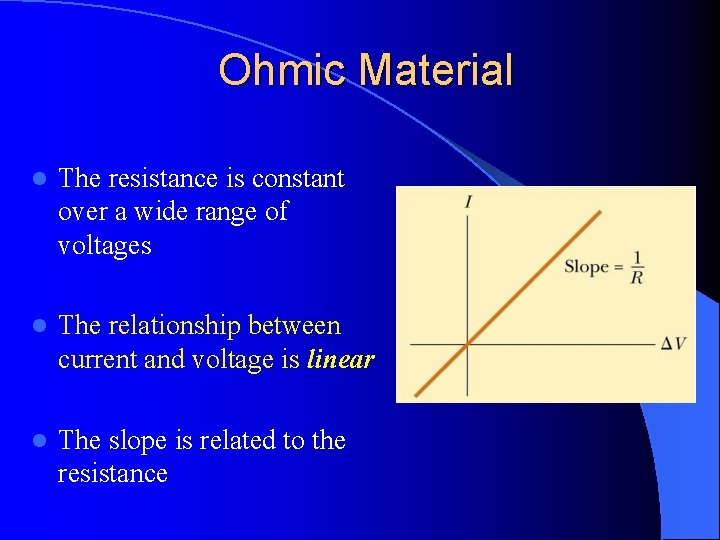Ohmic Material l The resistance is constant over a wide range of voltages l The relationship between current and voltage is linear l The slope is related to the resistance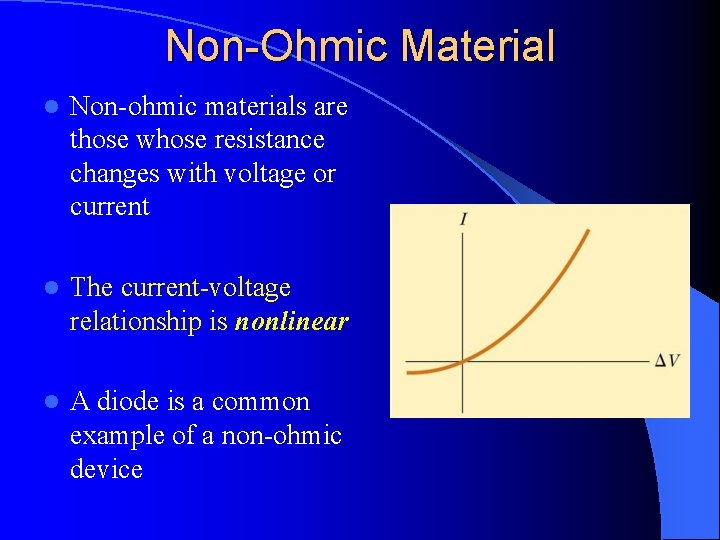Non-Ohmic Material l Non-ohmic materials are those whose resistance changes with voltage or current l The current-voltage relationship is nonlinear l A diode is a common example of a non-ohmic deviceQUICK QUIZ 17. 3 In the figure below, does the resistance of the diode (a) increase or (b) decrease as the positive voltage ∆V increases?QUICK QUIZ 17. 3 ANSWER (b) Decrease The slope of the line tangent to the curve at a point is the reciprocal of the resistance at that point. Note that as ΔV increases, the slope (and hence 1/R) increases. Thus, the resistance decreases.Resistors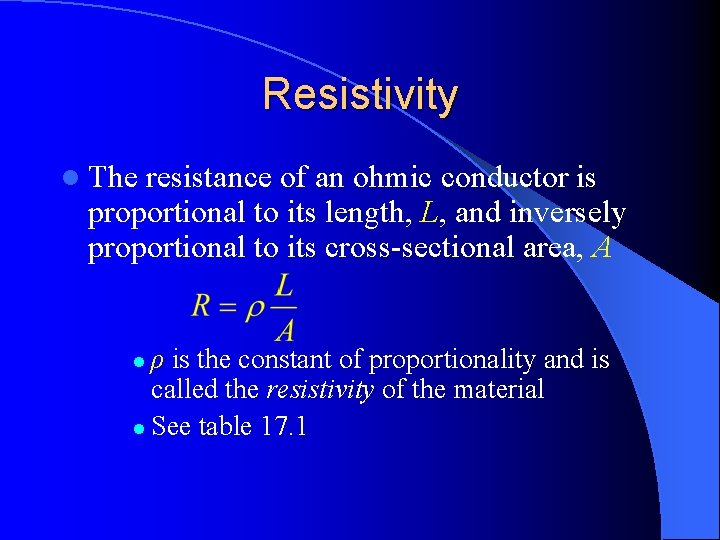Resistivity l The resistance of an ohmic conductor is proportional to its length, L, and inversely proportional to its cross-sectional area, A ρ is the constant of proportionality and is called the resistivity of the material l See table 17. 1 l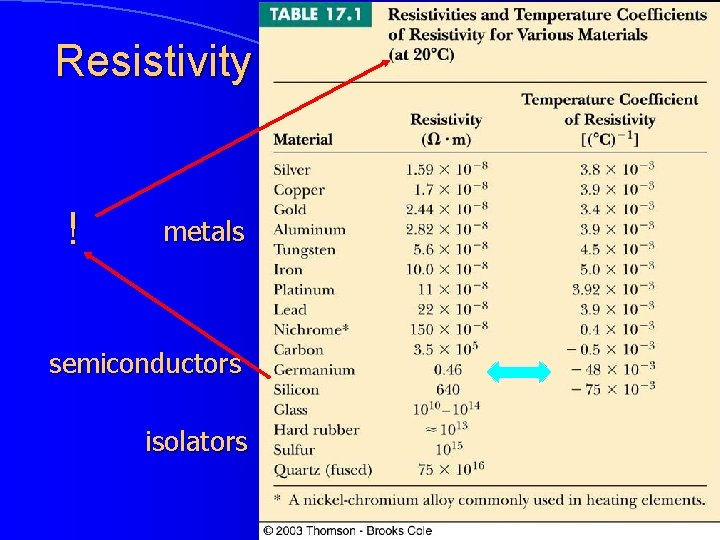Resistivity ! metals semiconductors isolatorsQUICK QUIZ 17. 4 Aliens with strange powers visit Earth and double every linear dimension of every object on the surface of the Earth. Does the electrical cord from the wall socket to your floor lamp now have (a) more resistance than before, (b) less resistance, or (c) the same resistance? Does the light bulb filament glow (d) more brightly than before, (e) less brightly, or (f) the same? (Assume the resistivities of materials remain the same before and after the doubling. )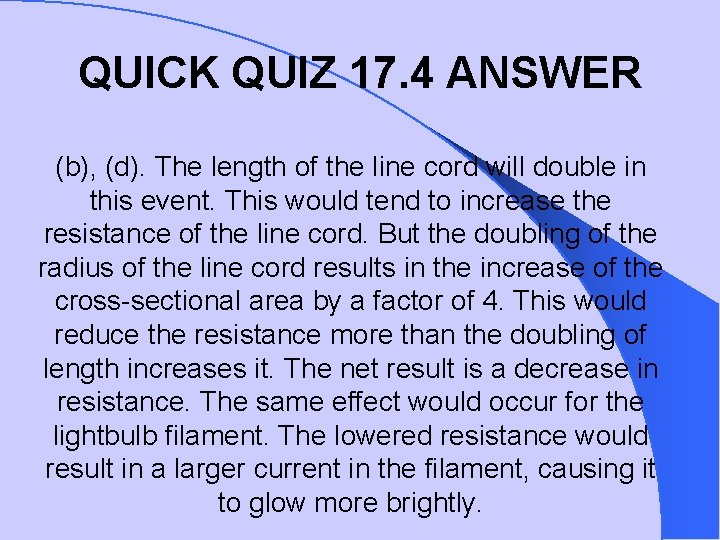QUICK QUIZ 17. 4 ANSWER (b), (d). The length of the line cord will double in this event. This would tend to increase the resistance of the line cord. But the doubling of the radius of the line cord results in the increase of the cross-sectional area by a factor of 4. This would reduce the resistance more than the doubling of length increases it. The net result is a decrease in resistance. The same effect would occur for the lightbulb filament. The lowered resistance would result in a larger current in the filament, causing it to glow more brightly.QUICK QUIZ 17. 5 A voltage V is applied across the ends of a nichrome heater wire having a cross-sectional area A and length L. The same voltage is applied across the ends of a second heater wire having a cross-sectional area A and length 2 L. Which wire gets hotter? (a) the shorter wire, (b) the longer wire, or (c) not enough information to say.QUICK QUIZ 17. 5 ANSWER (a). The resistance of the shorter wire is half that of the longer wire. The power dissipated, P = (ΔV)2/R, (and hence the rate of heating) will be greater for the shorter wire. Consideration of the expression P = I 2 R might initially lead one to think that the reverse would be true. However, one must realize that the currents will not be the same in the two wires.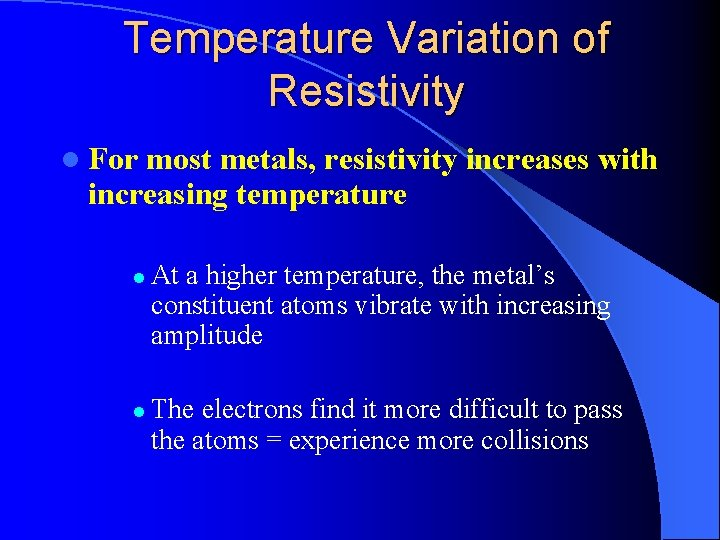Temperature Variation of Resistivity l For most metals, resistivity increases with increasing temperature l l At a higher temperature, the metal’s constituent atoms vibrate with increasing amplitude The electrons find it more difficult to pass the atoms = experience more collisionsTemperature Variation of Resistivity l For most metals, resistivity increases approximately linearly with temperature over a limited temperature range – ρo is the resistivity at some reference temperature To To is usually taken to be 20° C l is the temperature coefficient of resistivity l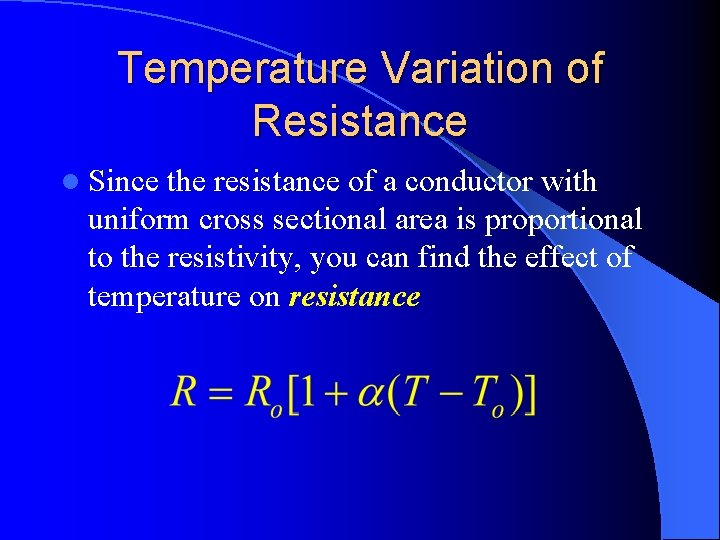Temperature Variation of Resistance l Since the resistance of a conductor with uniform cross sectional area is proportional to the resistivity, you can find the effect of temperature on resistance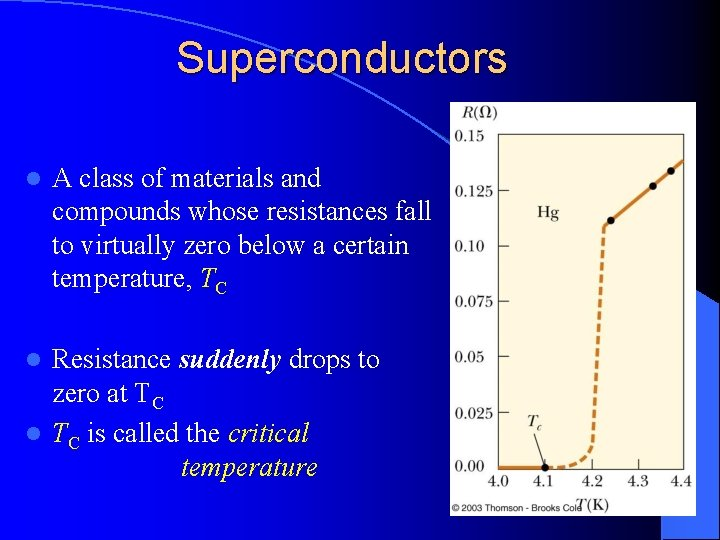Superconductors l A class of materials and compounds whose resistances fall to virtually zero below a certain temperature, TC Resistance suddenly drops to zero at TC l TC is called the critical temperature l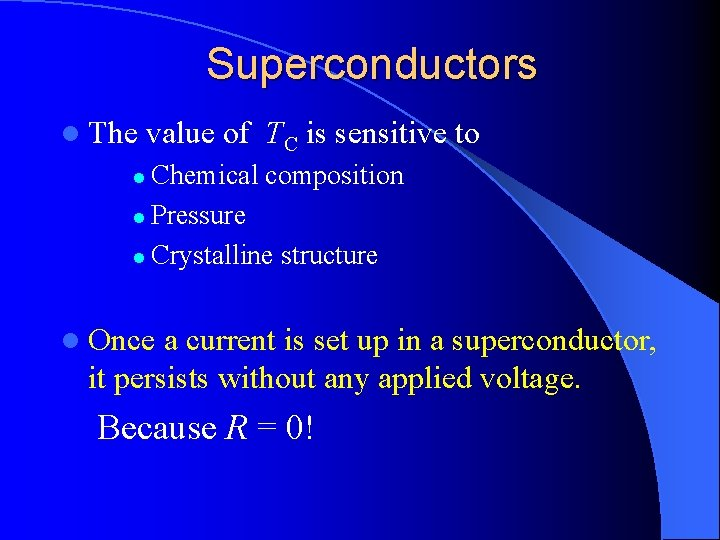Superconductors l The value of TC is sensitive to Chemical composition l Pressure l Crystalline structure l l Once a current is set up in a superconductor, it persists without any applied voltage. Because R = 0!Superconductor Timeline l 1911 l Superconductivity discovered by H. Kamerlingh Onnes l 1986 High temperature superconductivity discovered by Bednorz and Müller l Superconductivity near 30 K l l 1987 l Superconductivity at 96 K and 105 K l Current l More materials and, hopefully, more applications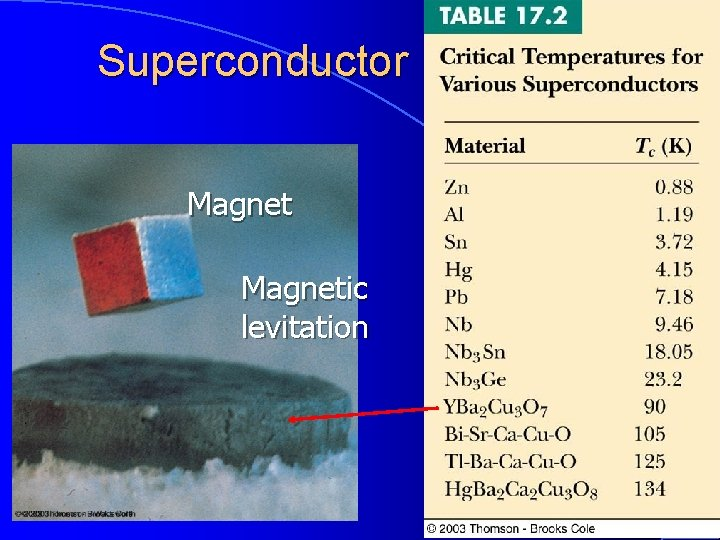Superconductor Magnetic levitation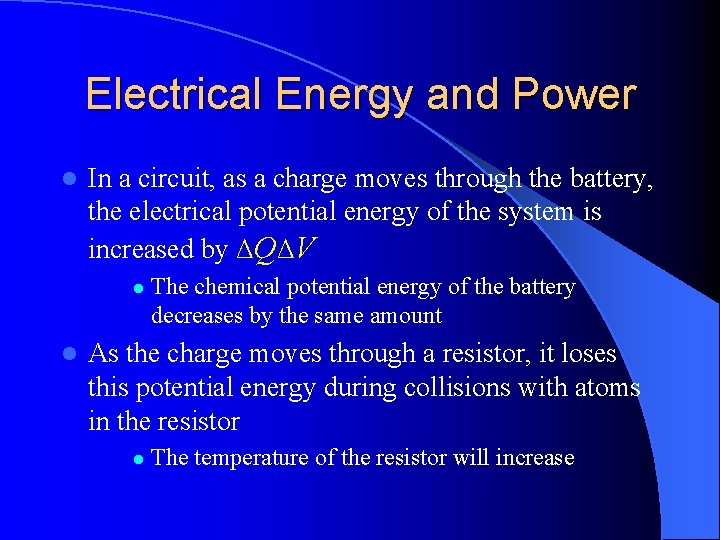Electrical Energy and Power l In a circuit, as a charge moves through the battery, the electrical potential energy of the system is increased by ΔQΔV l l The chemical potential energy of the battery decreases by the same amount As the charge moves through a resistor, it loses this potential energy during collisions with atoms in the resistor l The temperature of the resistor will increaseElectrical Energy and Power l The rate at which the energy is lost is the power l From Ohm’s Law, alternate forms of power are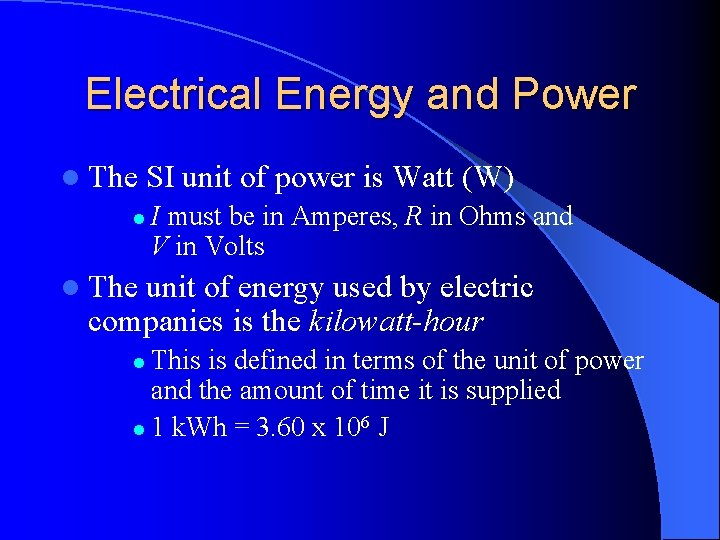Electrical Energy and Power l The SI unit of power is Watt (W) l I must be in Amperes, R in Ohms and V in Volts l The unit of energy used by electric companies is the kilowatt-hour This is defined in terms of the unit of power and the amount of time it is supplied l 1 k. Wh = 3. 60 x 106 J l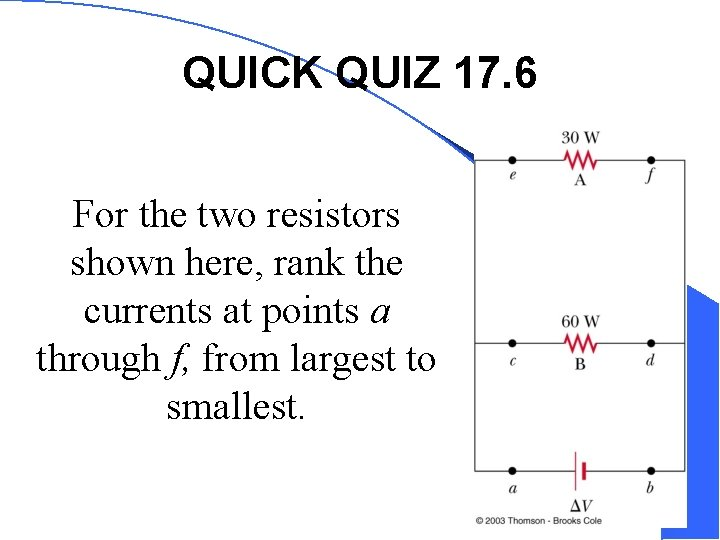QUICK QUIZ 17. 6 For the two resistors shown here, rank the currents at points a through f, from largest to smallest.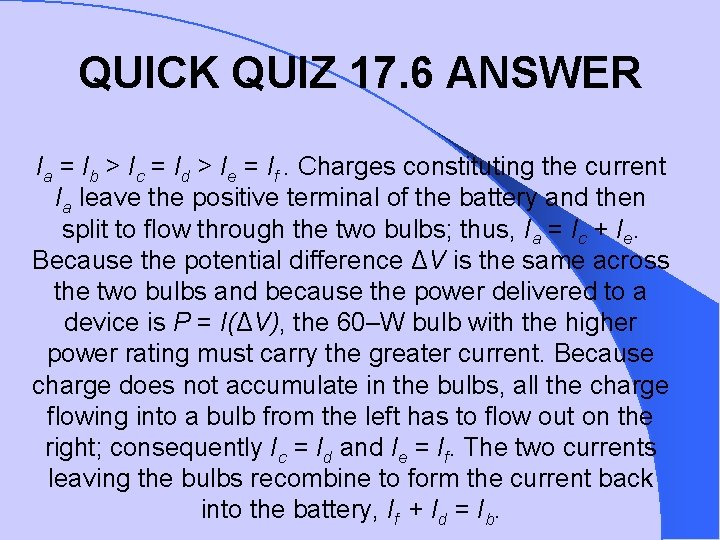QUICK QUIZ 17. 6 ANSWER Ia = Ib > Ic = Id > Ie = If. Charges constituting the current Ia leave the positive terminal of the battery and then split to flow through the two bulbs; thus, Ia = Ic + Ie. Because the potential difference ΔV is the same across the two bulbs and because the power delivered to a device is P = I(ΔV), the 60–W bulb with the higher power rating must carry the greater current. Because charge does not accumulate in the bulbs, all the charge flowing into a bulb from the left has to flow out on the right; consequently Ic = Id and Ie = If. The two currents leaving the bulbs recombine to form the current back into the battery, If + Id = Ib.QUICK QUIZ 17. 7 Two resistors, A and B, are connected across the same potential difference. The resistance of A is twice that of B. (a) Which resistor dissipates more power? (b) Which carries the greater current?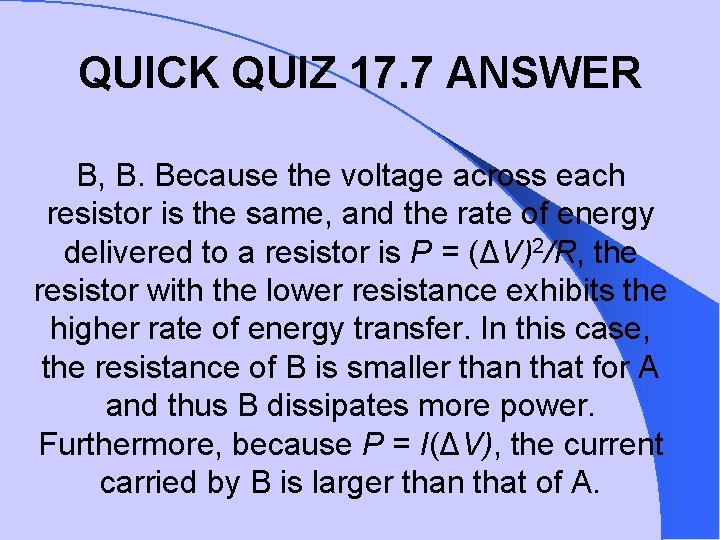QUICK QUIZ 17. 7 ANSWER B, B. Because the voltage across each resistor is the same, and the rate of energy delivered to a resistor is P = (ΔV)2/R, the resistor with the lower resistance exhibits the higher rate of energy transfer. In this case, the resistance of B is smaller than that for A and thus B dissipates more power. Furthermore, because P = I(ΔV), the current carried by B is larger than that of A.Electrical Activity in the Heart Every action involving the body’s muscles is initiated by electrical activity l Voltage pulses cause the heart to beat l These voltage pulses are large enough to be detected by equipment attached to the skin l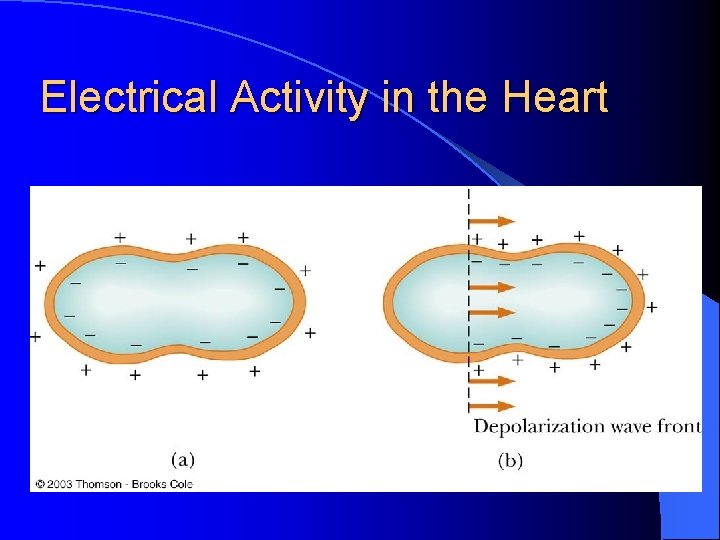Electrical Activity in the Heart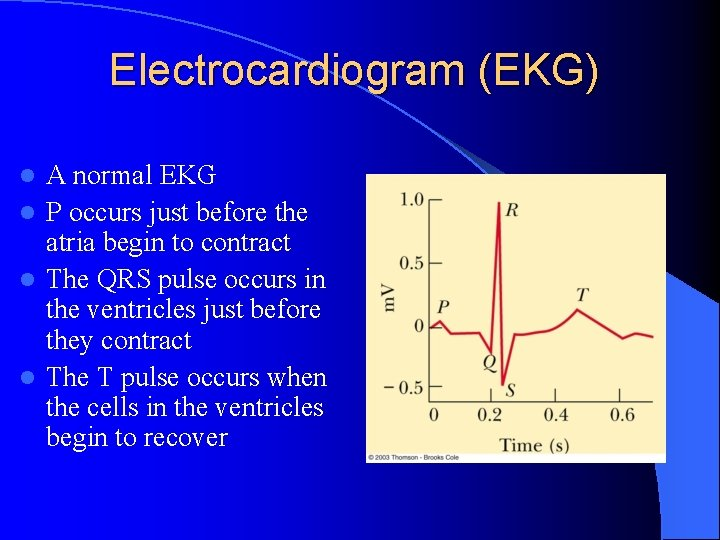Electrocardiogram (EKG) A normal EKG l P occurs just before the atria begin to contract l The QRS pulse occurs in the ventricles just before they contract l The T pulse occurs when the cells in the ventricles begin to recover l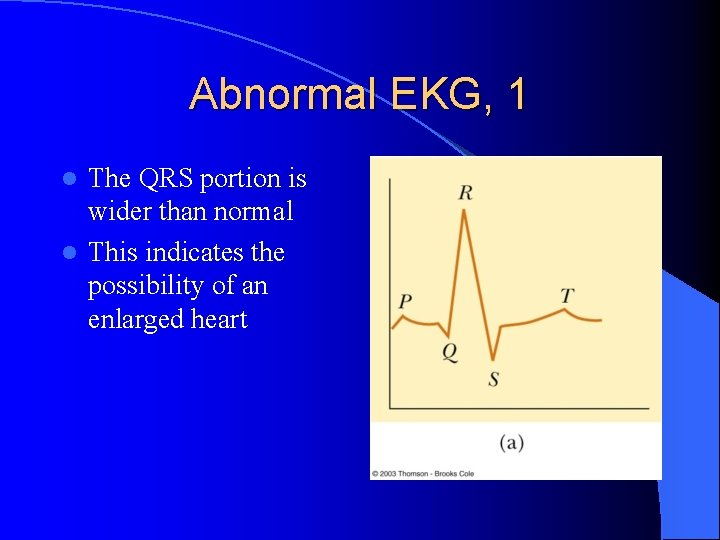Abnormal EKG, 1 The QRS portion is wider than normal l This indicates the possibility of an enlarged heart lAbnormal EKG, 2 There is no constant relationship between P and QRS pulse l This suggests a blockage in the electrical conduction path between the SA and the AV nodes l This leads to inefficient heart pumping lAbnormal EKG, 3 No P pulse and an irregular spacing between the QRS pulses l Symptomatic of irregular atrial contraction, called fibrillation l The atrial and ventricular contraction are irregular lImplanted Cardioverter Defibrillator (ICD) Devices that can monitor, record and logically process heart signals l Then supply different corrective signals to hearts that are not beating correctly l